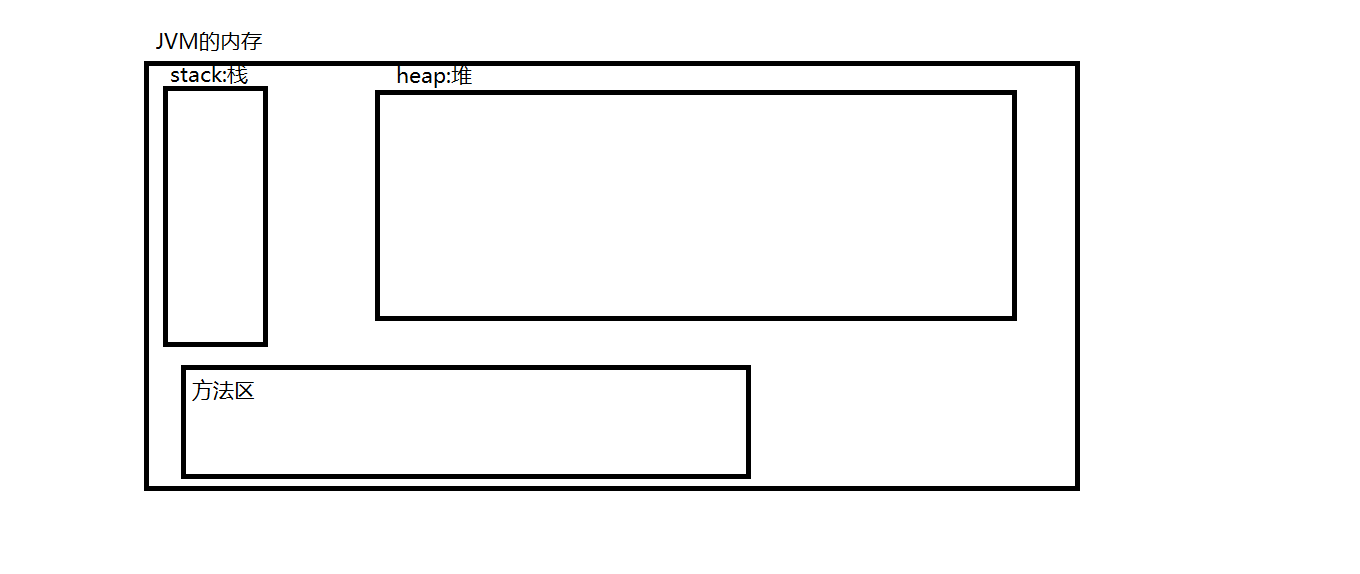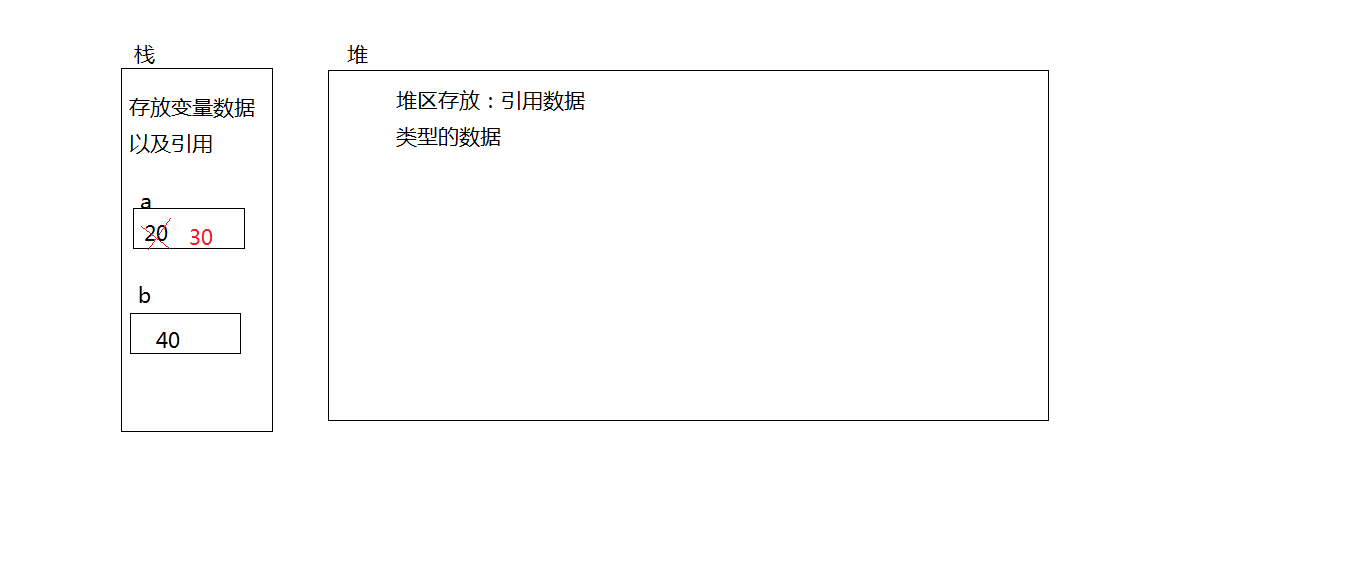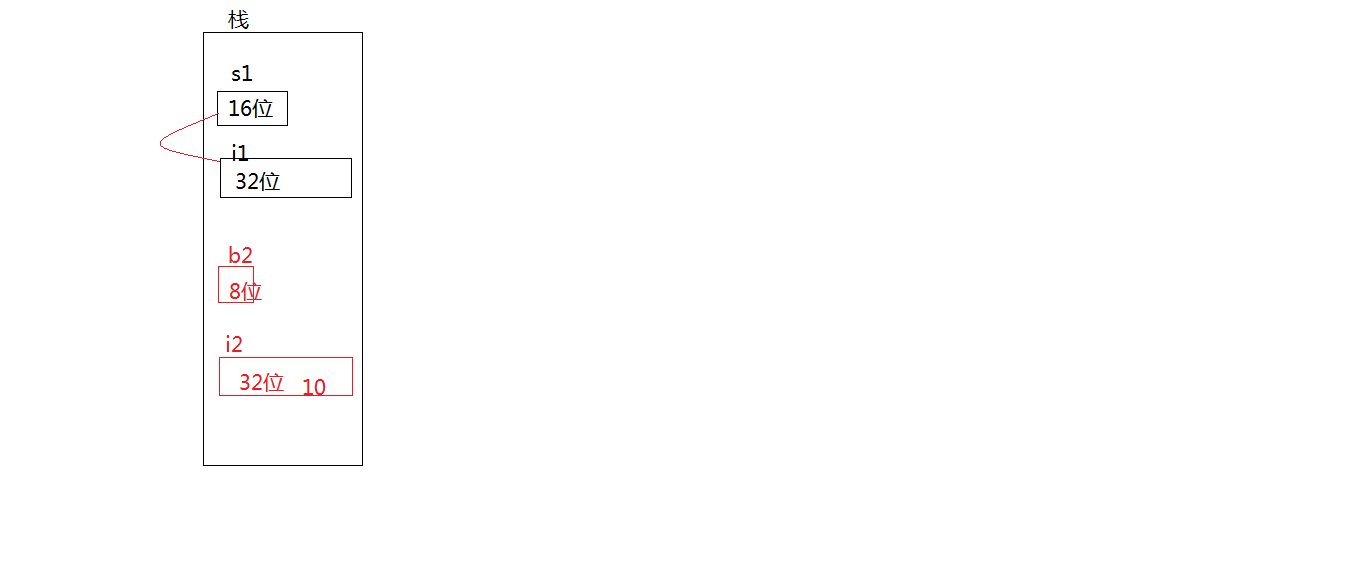1、关键字、保留字和标识符

• 关键字不能用作变量名、方法名、类名、包名
保留字：Java中还没有使用的英文单词，以后用不用不清楚。例如：goto...
标识符:java中包名、类名、方法名、变量名、常量名的总称

• 标识符的命名规则：

• 必须以字母、下划线（_）或美元符号（$）开头 • 余下的字符可以是下划线、美元符号或任何的字母或数字，长度不限； • 标识符中不能有空格 • 不能使用Java中的关键字或者保留字做为标识符； • 在同一个包中的类名不能相同 • 标识符的命名规范 • 包名：域名.公司名.项目名.种族-- 常见的域名： com、cn、org... com.neusoft.javase_day1.test • 类名：驼峰命名法 ，每个类名中的单词首字母要大写 StudentTestOne 或者Student_Test_One • 变量名、方法名、参数名：驼峰命名法-- 命名是首字母小写其他英文单词首字母大写 getAge () myAge • 常量名：要求所有的字母都大写，每个英文单词之间使用"_"隔开: MAX_AGE_MY /** * 文档注释 * @author Administrator * hellowordtest：包名 * HelloWorld:类名 * main：方法名 * args：参数名、变量名 */ public class HelloWorld { /** * main方法：程序的入口 * @param args :参数 */ public static void main(String[] args) { // 在控制台打印输出 /*在控制台打印输出 System.out.println("helloworld");*/ int i=10; // int 898=10; int j898=10; int$898=10;
int _898=10;
//      int @898=10;
//      int i j=10;

}

• 概念：程序中随时可能发生改变的量

• 变量是程序中数据的临时存放场所

• 保存程序运行时用户输入的数据
特定的运算结果等

• jvm内存• 变量内存/**
*  初始化 :第一次赋值
*  变量必须初始化之后才能使用
*所有的数据必须在内存中存在我们才能使用
* @param args
*/
public static void main(String[] args) {
//变量是指在程序的运行过程中随时可以发生变化的量

//变量的声明 int:数据类型 a:变量名  20:变量的值
int a;
//      System.out.println(a);
//变量的赋值：初始化
a=20;
System.out.println(a);//20
a=30;
System.out.println(a);//30
//变量的声明同时初始化
int b=40;
System.out.println(b);//40
}

JAVA中的数据类型：

• 数值型：

• 整型：byte、short、int、long

public static void main(String[] args) {
//整型：byte、short、int、long
// 1G=1024m  1m=1024k  1k=1024bit
// byte :字节     1字节=8bit  1字节  8位   -2^7~2^7-1
byte b1=20;
//      byte b2=128;
System.out.println(b1);

//short:2字节 16位   -2^15~2^15-1
short s1=12312;

//int:4字节 32位  -2^31~2^31-1
//**注意：java中默认的整型为int
int i1=13271;

//long:8字节   64位
//注意：long类型的数据需要在其后面加上“L”
long l1=1234;// int --long
long l2=1234L;

//十进制：不能以0开头
int i2=01312;
System.out.println(i2);//714
i2=5464;
System.out.println(i2);//5464

//八进制：必须以0开头
int i3=013121; // 1*8^0+2*8^1+1*8^2+3*8^3+1*8^4=5713
System.out.println("i3:"+i3);//i3:5713

//十六进制：必须以0x开头  后面的数据只能是0 ~ 9  A ~ F
int i4=0xba3;// 3*16^0+10*16+11*16^2
System.out.println("i4:"+i4);//i4:2979

//二进制：必须以0b开头
int i5=0b01010110; //0*2^0+1*2^1.....
System.out.println("i5:"+i5);//i5:86
// jdk1.7之后使用“_”提高代码的可阅读性
i5=0b1010_0101_1111;
System.out.println("i5:"+i5);//i5:2655
• 浮点型：float、double

      //  浮点型：float、double
//      float :4字节 32位   值后面需要加F或f
float f1=1234.5F;
//      float f2=1234.5;
//      double:8字节 64位:
//      double是java中默认的浮点型
double d1=12345.567;
double d2=12345.567d;

//char:字符类型
//①接收字符，表示的是单个字符，只能接收一个字符
char c1='中';
System.out.println(c1);//中
//②接收整型，最大2字节16位 表示的是：JVM会去查找ascii码表数值对应的字符
char c2=49;
char c3=65;
System.out.println("c2:"+c2+" c3:"+c3);//c2:1 c3:A
char c4=97;
System.out.println("c4:"+c4);//c4:a

//③接收Uncode字符  以\ u开头的字符：JVM会去查找Uncode码表数值对应的字符
char c5='\u03A6';
System.out.println(c5);//Φ

//④接收16进制就是接收整型：将16进制转为10进制再对应的ascii码值
char c6=0x23;
System.out.println(c6);//#

//      转义字符：使用”\”把其转化成字符的本身输出，那么使用”\”的字符称作为转义字符。
System.out.println("\"");//"
System.out.print("你好");//不换行输出
System.out.println("\n");//换行
System.out.print("中国");//你好中国
System.out.println("在吗");
System.out.println("中国：在");
//你好”中国”
System.out.println("你好\"中国\"");//你好"中国"


//布尔型：false true
boolean temp=false;
boolean temp1=true;
//      boolean temp2=1;
System.out.println(temp);//false
• 基本数据类型的转换/**
*  精髓：看数据类型精度的大小
*     浮点型比整型大
*
*/
public class _基本数据类型转换 {

public static void main(String[] args) {
//隐式转换 ：小精度--大精度
short s1=321;
int i1=s1;
System.out.println("i1:"+i1);//i1:321

byte b1=23;
short s2=b1;
System.out.println("s2:"+s2);//s2:23

//      int和float之间转换：int--float
float f1=i1;

//long<float
float f3=1231L;
double d1=123L;
System.out.println("f3:"+f3);//f3:1231.0
System.out.println("d1:"+d1);//d1:123.0
//显示转换(强制转换) :强制转换，大精度--小精度,需要注意当大精度数据中的值大于小精度能够接受的最大范围会出现精度丢失
int i2=10;
byte b2=(byte) i2;
System.out.println(b2);//10
int i3=129;
byte b3=(byte)i3;
System.out.println(b3);//-127

//double --float
float f2=(float) 123.45;
System.out.println(f2);//123.45

//float--int
int i4=(int) 1945.5F;
int i5=(int) 1945.9F;
System.out.println(i4);//1945
System.out.println(i5);//1945

// 算术运算符 +
int m=10;
int n=m+10;
System.out.println(n);
//不同基本数据类型进行计算时，会先转为其中最大精度的数值类型再做计算
double dd1=123.3F+123+1.0;
int ii1=(int) (123.3F+123+1.0);

//在计算时byte、short、char之间不会相互转换，他们三者在计算时首先会转换为int类型。
byte bb1=90;
short ss1=80;
short ss2=(short) (bb1+ss1);
int ii2=bb1+ss1;

int c0='A';
System.out.println(c0);//65
int ii3='B'+bb1+ss1;
System.out.println(ii3);//236

//int--char
int ii4=56;
char c1=(char) ii4;
System.out.println(c1);//8
System.out.println((char)ii4);//8
}


/**
*
*   计算机在存储数值类型数据时是以补码的形式存储的，读取显示时将补码转为原码输出
*  针对于二进制: 第一位为符号位：1表示负数  0表示整数
* 正数的原码、反码、补码都一样
*负数： 反码：原码除了符号位不变之外其余取反    补码=反码+1
*    补码转原码：补码-1然后除了符号位以外其余取反
*
*/
public class _原码反码补码 {
public static void main(String[] args) {
//正数的原码、反码、补码都一样
int i=129;//   0000 0000 0000 0000 0000 0000 1000 0001
int i1=-129;// 1000 0000 0000 0000 0000 0000 1000 0001

byte b2=5;//原码：0000 0101  反码：0000 0101  补码：0000 0101
byte b3=-5;//原码1000 0101  反码：1111 1010  补码：1111 1011

byte b4=(byte) 129;// 补码：1000 0001  反码：1000 0000 原码：1111 1111
System.out.println(b4);//-127

System.out.println(0b01111111);//127
System.out.println((byte)0b11111111);//-1 1111 1111为补码，会将补码转为原码再显示

}
}
• 赋值运算符


//        赋值运算符：=、+=、﹣=、*=、/=、%=   从右往左，优先级最低
int a=20;
int a1=10+20;

a+=10;  // a=a+10
System.out.println(a);//30
a/=5;// a=a/5;
a%=4;
System.out.println(a);//2
• 算术运算符


//         算术运算符： +、﹣、*、/、%、++、 --
//        /求整：求两个数相除之后整数部分，符号负负得正
int b=15/-6;
System.out.println(b);//-2
//        %求余：求两个数相除之后余数部分，符号与被除数相同
b=15%-6;
System.out.println(b);//3
• 比较运算符


//        比较运算符：>、<、>=、 <=、= =、!=、instanceof:返回boolean类型的数据
boolean t1=1<2;
System.out.println(t1);//true
//        ==用于比较引用数据类型时比较的是两个对象的地址(后续详细讲解)
//        ==用于比较基本数据类型时比较的是两个值的大小
boolean t2=67==78;
System.out.println(t2);//false
//        != 判断两个值是否不相等，如果不相等返回true 否则返回false
t2=67!=68;
System.out.println(t2);//true
//        instanceof 只能比较引用数据类型，用于判断某个对象是否是属于某一类型
Date d=new Date();
boolean t3=d instanceof Date;
System.out.println(t3);//true
• 位运算


//        位运算符： &、|、^ 、 ~、>>、<<、>>>  按位运算  只对byte、short、char、int、long有效
//       &按位与  只有参加运算的两位都为1，&运算的结果才为1，否则为0
int i1=9&3;
/*
* 0000 1001
0000 0011
0000 0001
*/
System.out.println(i1);//1

//      | 按位或 只有参加运算的两位都为0，|运算的结果才为0，否则为1
i1=9|3;
/*
* 0000 1001
0000 0011
0000 1011
*/
System.out.println(i1);//11
//      ^ 异或 只有参加运算的两位不同，^运算的结果才为1，否则为0
i1=9^3;

/*
* 0000 1001
0000 0011
0000 1010
*/
System.out.println(i1);//10

//    <<  左移 a<<b,将a的二进制数据左移b位，右边移空的部分补0   ：移动的是补码
//    ①m正数左移：m<<n   m*2的n次幂
//    0000 0000 1001
i1=9<<2;//0000 0010 0100
System.out.println(i1);//36
i1=9<<3;//0000 0100 1000
System.out.println(i1);//72

//    ②m负数左移m<<n   m*2的n次幂
i1=-9<<2;
System.out.println(i1);//-36
i1=-4<<3;
System.out.println(i1);//-32

//    >> 右移 a>>b,将a的二进制数据右移b位，如果最高位是0，则左边移空的部分补0，如果最高位是1，则左边移空的部分补1 移动的是补码
//    ①m正数右移   m>>n m/2的n次幂
//    0000 0000 1001
i1=9>>2;//0010
System.out.println(i1);//2
i1=9>>3;//0001
System.out.println(i1);//1
i1=9>>1;
System.out.println(i1);//4

//      ②负数右移：
//      1 101101 --反码：1 010010   补码 1 010011   右移： 1 110100
//      补码： 1 110100 反码1 110011  原码：1 001100
i1=-45>>2;
System.out.println(i1);//-12
System.out.println(-45/4);

// 按位与和按位或都有逻辑功能
//    逻辑与和按位与的区别：他们都有逻辑功能,
//    a&&b 逻辑与有短路功能如果知道a为false,b不执行，整个表达式为false
//    a&b 按位与，没有短路功能如果知道a为false,b继续执行，整个表达式为false，也就是无论如何表达式b都会执行
int p=10;
boolean temp1=p<9 && p++<10;
System.out.println(p);//10
temp1=p<9 & p++<10;
System.out.println(p);//11

//    逻辑或和按位或得区别：他们都有逻辑功能
//    a||b  逻辑或有短路功能，如果知道a为true,b不执行，整个表达式为true
temp1=p<14 || p++<10;
System.out.println(p);//11
//    a|b  按位或没有有短路功能，如果知道a为true,b继续执行，整个表达式为true，也就是无论如何表达式b都会执行
temp1=p<14 | p++<10;
System.out.println(p);//12
• 三元表达式
//     条件运算符： ?：   语法：   a?b:c  如果a为true返回b否者返回c  相当于if(){}else{}   从右往左运算
//    0:男    1：女
char c='男';
int sex=c=='男'?0:1;
System.out.println(sex);//0

boolean k=false?true:false?false:false?true:false?true:true;
System.out.println(k);//true

• 运算符的优先级
//    运算符的优先级：括号的优先级最高
//    表达式：运算符+运算数
//    按操作数多少划分：一元操作符 > 二元操作符 > 三元操作符 :先计算1+2 和 1+1 再计算1+2<5 && 1+1==2  左后才是三元表达式
String ss1=1+2<5 && 1+1==2 ?"是的":"不是的";
//    按运算类型划分
//    算术运算符 > 关系运算符 > 逻辑运算符 > 赋值运算符
int ii1=1+2+3;//6
int ii2=ii1++/--ii1;
System.out.println(ii2);//1